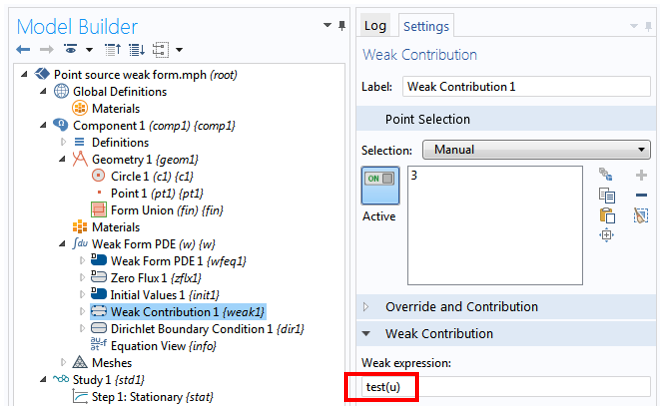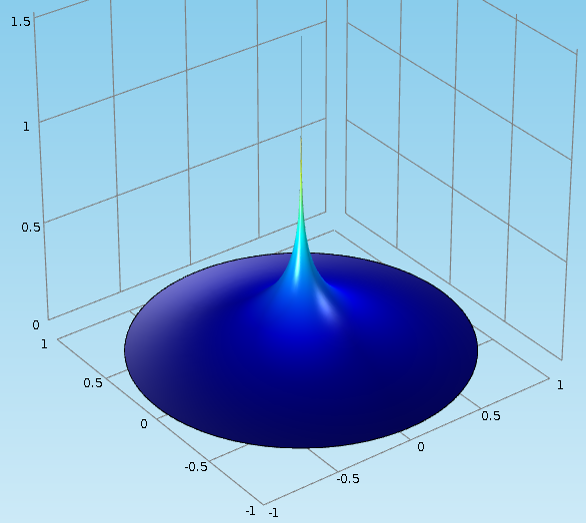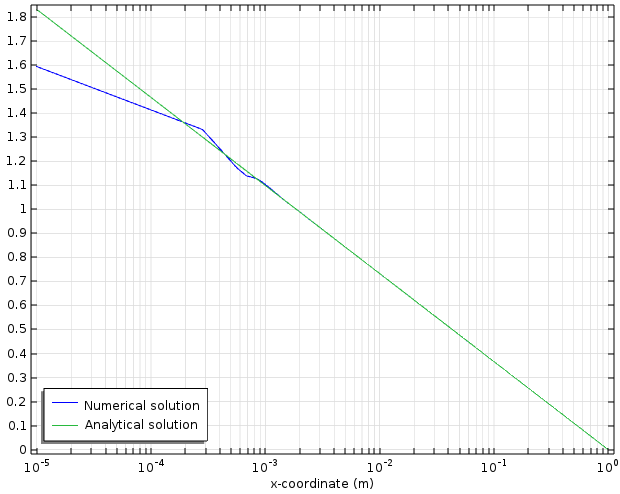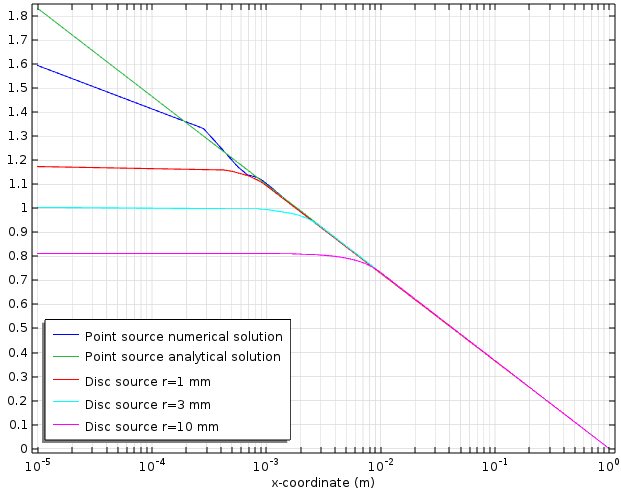# 如何用弱形式实现点源

2015年 8月 24日

### 点源的数学基础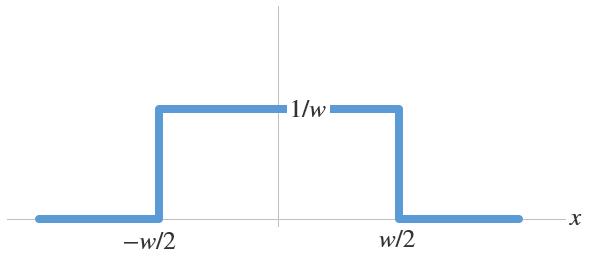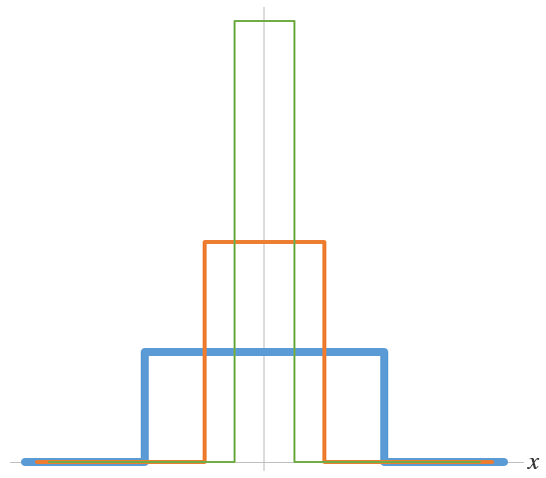δ 函数有一些方便的特性。除了原点，它的值在任何地方都是零。

\delta(x)= \left\{ \begin{array}{ll}
\infty \mbox{ for } x=0\\
0 \mbox{ elsewhere}
\end{array} \right.

\int^\epsilon_{-\epsilon} \delta(x) f(x) dx=f(0) \mbox{ for all } \epsilon > 0

\delta(x-a)= \left\{ \begin{array}{ll}
\infty \mbox{ for } x=a\\
0 \mbox{ elsewhere}
\end{array} \right.

\int^{a+\epsilon}_{a-\epsilon} \delta(x-a) f(x) dx=f(a) \mbox{ for all } \epsilon > 0

\delta(x-a,y-b)= \delta(x-a)\delta(y-b) = \left\{ \begin{array}{ll}
\infty \mbox{ for } x=a, y=b\\
0 \mbox{ elsewhere}
\end{array} \right.

(1)

\iint\limits_{\Omega} \delta(x-a,y-b) f(x,y) dx dy=f(a,b) \mbox{ for all } \Omega \ni (a,b)

### 使用弱形式实现点源

(2)

-\left({\partial^2 \over \partial x^2}+{\partial^2 \over \partial y^2} \right)u(x,y) = \delta(x,y)

(3)

\iint\limits_{\Omega} \delta(x,y) \tilde{u}(x,y) dx dy=\tilde{u}(0,0)